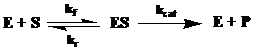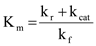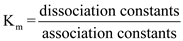Biology

## Quiz 5 : EnzymesLooking for Biotechnology Homework Help?# Quiz 5 : Enzymes

The enzyme catalyzed reaction can be stated as follows:Where k f = forward rate constant for the formation of ES (enzyme substrate complex) from E (enzyme) and S (substrate). k r = reverse rate constant for the formation of E and S from ES k cat = the rate constant for the breakdown of ES to E and P (product)From this reaction, the K m (rate constant) is as follows:Thus, the value of Km would be equal to dissociation constant when the association constants would be 1. The Km is the constant which is the ratio of dissociation constant to the association constant; whereas the dissociation constant is the sum of constants for the breakdown of ES (enzyme substrate complex), either to E and S or E and P.

K m is the substrate concentration at which the velocity of an enzyme action is half that of its maximum velocity. K m and substrate concentration have same units. However, biochemically, K m is the ratio of the rate constants leading to the breakdown of the ES complex to the rate constant leading to the formation of the ES complex. In a cell, the concentration of an enzyme's substrate is almost equal to the K m of the enzyme. This ensures that by increasing or decreasing the substrate concentration, the velocity of enzyme reaction can be varied. In short, this is the region where the enzyme regulation can be at its maximum rate, even by making small changes in the substrate concentration.

Vmax is defined as the maximum velocity at which the enzyme can function to its best. Each enzyme has its own and different Vmax values. This is because each enzyme has different binding capacity with the different substrates. Sometimes, same enzyme can show different Vmax with different substrates. The enzyme action is based upon the formation of substrate enzyme complex. This complex formation does not require same amount of energy for binding thus, each enzyme has different Vmax.